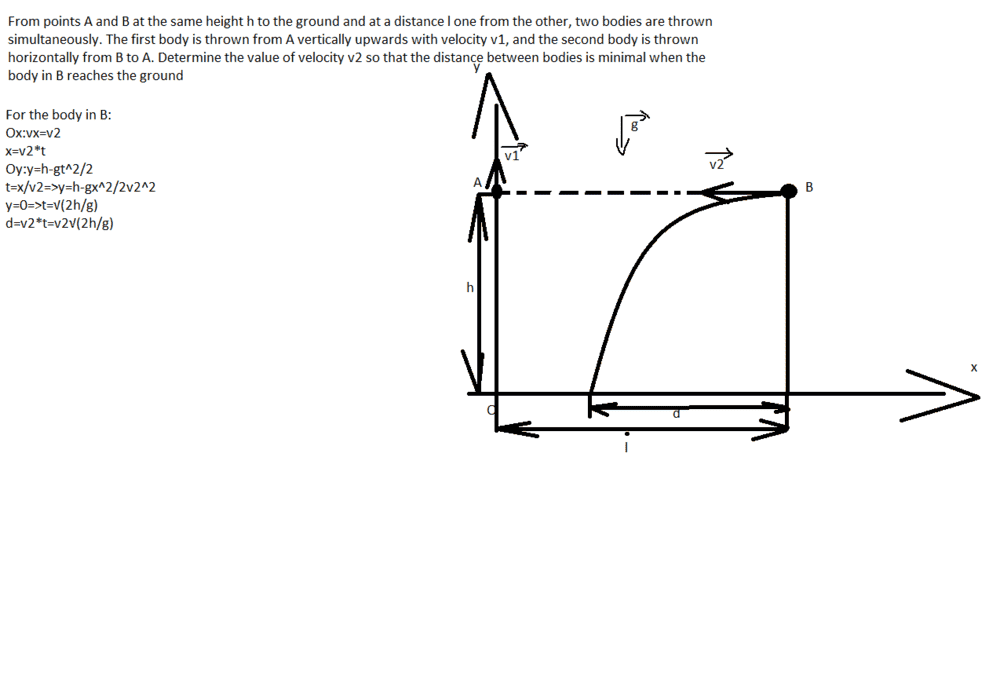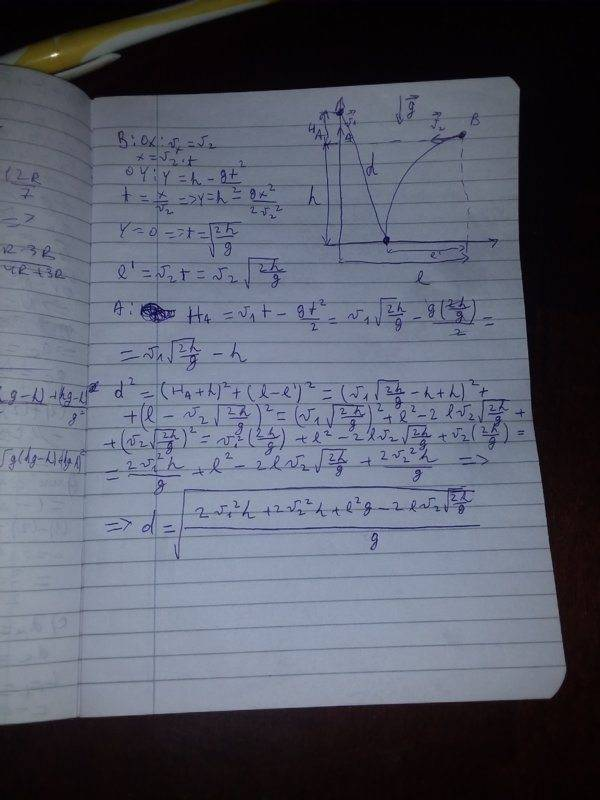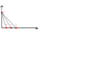# Mechanics problem — One body thrown vertically and one thrown horizontally

Baiatul122001
New user has been reminded to show their work on schoolwork questions
Homework Statement:
Find out the speed of the body B
Relevant Equations:
From here you continue, which is the minimum condition, pleaseLast edited by a moderator:

Homework Helper
Gold Member
2022 Award
Homework Statement:: Find out the speed of the body B
Homework Equations:: From here you continue, which is the minimum condition, please

We can only help with your homework, not do it for you. You should give this question your best attempt post again if you get stuck, showing us how much you've done.

Homework Helper
Gold Member
2022 Award
So where is the body thrown from A at that time you calculated?

Baiatul122001
It's in the figure next to where I got stuck

Baiatul122001
I do not know if he has reached the maximum height or has continued to reach the maximum height

Homework Helper
Gold Member
2022 Award
It's in the figure next to where I got stuck
I meant as an algebraic expression.

Gold Member
2022 Award
Your v1 and v2 seem to bear no relationship to the actual problem statement.

•archaic
lightlightsup
I took the time it takes object B to fall to the ground and I used it to calculate object A's position along the vertical axis.
I used this time-for-B-to-hit-the-ground to calculate the distance between the 2 objects at that time (via Pythagorean Theorem).
The answer checked out for me, with and without calculus.

Here it is:
Hopefully, someone can try to double check me:
##v_2 = I\sqrt{\frac{g}{2h}}##

Last edited:
•PeroK
Homework Helper
Gold Member
2022 Award
We have to calculate the speed v2 so that the distance between the two is minimal. The answer must be:

Let's suppose first that ##v_1 = 0##. Now, the two objects fall together and for a range of speeds ##v_2## the objects will come closest together when they both hit the ground. In this case, therefore, there is no unique solution.

There must be a minimum value of ##v_1## for there to be a solution.

Let's suppose next that ##v_1## is large. Object A is fired up at high speed and the point at which they are closest is at ##t=0##.

There must be a maximum value of ##v_1## for there to be a solution.

Baiatul122001
During t the body from A climbs: H=v1t-gt^2/2=v1√(2h/g)-h/g.
So the height at which the body in A when the one in B reaches the ground is H'=H+h=v1√(2h/g)-h/g+h=v1√(2h/g)+(-h+hg)/g.
The horizontal distance between the bodies at this time is D=l-d=l-v2√(2h/g).
Now we apply the Pythagorean Theorem to find the distance between bodies:L^2=D^2+H'^2=[l-v2√(2h/g)]^2+[v1√(2h/g)+(-h+hg)/g]^2=l^2-2l√(2h/g)v2+(2hv2^2+2hv1^2)/g+2√2v1√h√g(hg-h)/g^2+(hg-h)^2/g^2.
Consider the function f (v2) =l^2-2l√(2h/g)v2+(2hv2^2+2hv1^2)/g+2√2v1√h√g(hg-h)/g^2+(hg-h)^2/g^2 , do we calculate the minimum of the function?

Homework Helper
Gold Member
2022 Award
During t the body from A climbs: H=v1t-gt^2/2=v1√(2h/g)-h/g.
So the height at which the body in A when the one in B reaches the ground is H'=H+h=v1√(2h/g)-h/g+h=v1√(2h/g)+(-h+hg)/g.
The horizontal distance between the bodies at this time is D=l-d=l-v2√(2h/g).
Now we apply the Pythagorean Theorem to find the distance between bodies:L^2=D^2+H'^2=[l-v2√(2h/g)]^2+[v1√(2h/g)+(-h+hg)/g]^2=l^2-2l√(2h/g)v2+(2hv2^2+2hv1^2)/g+2√2v1√h√g(hg-h)/g^2+(hg-h)^2/g^2.
Consider the function f (v2) =l^2-2l√(2h/g)v2+(2hv2^2+2hv1^2)/g+2√2v1√h√g(hg-h)/g^2+(hg-h)^2/g^2 , do we calculate the minimum of the function?

To be honest, I'm not sure how to interpret this problem. It may be more complicated than it was supposed to be. I don't have time to look at it carefully, but something doesn't seem quite right. See my post above if ##v_1 =0## or if ##v_1## is small.

Baiatul122001
For v1 >> 0, the point where they are closest is at t = 0, ie when the bodies are in the initial position have the minimum distance between them?

•PeroK
Homework Helper
Gold Member
2022 Award
During t the body from A climbs: ##H=v_1t-gt^2/2=v_1√(2h/g)-h/g##.
So the height at which the body in A when the one in B reaches the ground is ##H'=H+h=v_1√(2h/g)-h/g+h=v_1√(2h/g)+(-h+hg)/g##
The horizontal distance between the bodies at this time is ##D=l-d=l-v_2√(2h/g)##
Now we apply the Pythagorean Theorem to find the distance between bodies:##L^2=D^2+H'^2=[l-v_2√(2h/g)]^2+[v_1√(2h/g)+(-h+hg)/g]^2=l^2-2l√(2h/g)v_2+(2hv_2^2+2hv_1^2)/g+2√2v_1√h√g(hg-h)/g^2+(hg-h)^2/g^2##
Consider the function ##f (v_2) =l^2-2l√(2h/g)v_2+(2hv_2^2+2hv_1^2)/g+2√2v_1√h√g(hg-h)/g^2+(hg-h)^2/g^2## , do we calculate the minimum of the function?
##v_1√(2h/g)-h/g## cannot be right. The left hand term is a distance, the right hand term is time2.
Similarly I see terms (hg-h) later, which make no sense. You should always check for dimensional consistency.

But as @lightlightsup notes, there is a much easier way to solve this.

For v1 >> 0, the point where they are closest is at t = 0, ie when the bodies are in the initial position have the minimum distance between them?
Yes, but that is irrelevant. You are only concerned with minimising the distance when B lands.
Let's suppose first that ##v_1 = 0##. Now, the two objects fall together and for a range of speeds v2 the objects will come closest together when they both hit the ground. In this case, therefore, there is no unique solution.
I think you are reading it as "find the value of v2 such that, of the distances between them during the motion, the minimum occurs when B hits the ground."
@lightlightsup and I read it as "of all the distances between them that might be when B hits the ground, find the value of v2 that minimises it."

Last edited:
•PeroK
Baiatul122001
##v_1√(2h/g)-h/g## cannot be right. The left hand term is a distance, the right hand term is time2.
Similarly I see terms (hg-h) later, which make no sense. You should always check for dimensional consistency.

It is squared because L ^ 2 = l ^ 2-2l√ (2h / g) v2 + (2hv2 ^ 2 + 2hv1 ^ 2) / g + 2√2v1√h√g (hg-h) / g ^ 2 + ( HG-h) ^ 2 / g ^ 2. It makes sense if we apply the radical, L = √[l ^ 2-2l√ (2h / g) v2 + (2hv2 ^ 2 + 2hv1 ^ 2) / g + 2√2v1√h√g (hg-h) / g ^ 2 + ( HG-h) ^ 2 / g ^ 2].
If we consider the function f (v2) =√[l ^ 2-2l√ (2h / g) v2 + (2hv2 ^ 2 + 2hv1 ^ 2) / g + 2√2v1√h√g (hg-h) / g ^ 2 + ( HG-h) ^ 2 / g ^ 2] ,how do we find the minimum?

Homework Helper
Gold Member
2022 Award
It is squared because
No matter what your reasoning, terms like (hg-h) are nonsensical. You cannot subtract a distance from the square of a velocity.

Baiatul122001
So it's not correct with functions, ahhh

Homework Helper
Gold Member
2022 Award
So it's not correct with functions, ahhh
Check how you got the h/g at the end of this:
##H=v_1t-gt^2/2=v_1√(2h/g)-h/g##.

Baiatul122001
Check how you got the h/g at the end of this:
##H=v_1t-gt^2/2=v_1√(2h/g)-h/g##.

I used the time obtained for the body from B to touch the ground and using the law of uniform rectilinear motion=v1t-gt^2/2=v1√(2h/g)-g[√(2h/g)]^2/2=v1√(2h/g)-g(2h/g)/2=v1√(2h/g)-h

Ahh, I was wrong the first time

Homework Helper
Gold Member
2022 Award
Check how you got the h/g at the end of this:
##H=v_1t-gt^2/2=v_1√(2h/g)-h/g##.

I used the time obtained for the body from B to touch the ground and using the law of uniform rectilinear motion=v1t-gt^2/2=v1√(2h/g)-g[√(2h/g)]^2/2=v1√(2h/g)-g(2h/g)/2=v1√(2h/g)-h

Ahh, I was wrong the first time
As I wrote, always check your final equation for dimensional consistency. If it is wrong, backtrack ("binary chop").

Homework Helper
Gold Member
2022 Award
I think you are reading it as "find the value of v2 such that, of the distances between them during the motion, the minimum occurs when B hits the ground."
@lightlightsup and I read it as "of all the distances between them that might be when B hits the ground, find the value of v2 such that minimises it."
That's why I prefer defining things properly instead of wordy descriptions. In this case:

Let ##d## be the distance between A and B when B hits the ground. For a given ##v_1## find ##v_2## such that ##d## is minimised.

Then all is clear.

Baiatul122001
Let ##d## be the distance between A and B when B hits the ground. For a given ##v_1## find ##v_2## such that ##d## is minimised.

What is the expression of "d" before minimization?

Homework Helper
Gold Member
2022 Award
Let ##d## be the distance between A and B when B hits the ground. For a given ##v_1## find ##v_2## such that ##d## is minimised.

What is the expression of "d" before minimization?

That's what you have to calculate.

Baiatul122001That's what you have to calculate.
[/QUOTE]

archaic
Suppose we are at ##t=\tau## when the body B reached the ground. How can you find ##\tau##?
Calculus approach:
How can you express the distance between both objects at that time, i.e ##d(\tau)##?
Your condition is on ##v_2##, so you want to minimize ##d(\tau)=f(v_2)##. What does that mean?
Algebra approach:
Think of it like this: B is on the ground, it doesn't matter where A is but it must be on the ##y##-axis. Where do you think B should be on the ##x##-axis for the distance between it and A to be minimum?Last edited:
•PeroK
Homework Helper
Gold Member
2022 Award
View attachment 254995
That's what you have to calculate.

Even if you don't use the short cut mentioned in the previous post, it shouldn't be very complicated.

B always takes the same time to reach the ground.

archaic
We have to calculate the speed v2 so that the distance between the two is minimal. The answer must be:
Is this the textbook's solution? Weird, my solution doesn't depend on ##v_1## at all, rather on ##h,\,g## and ##x_B(t=0s)##.

•neilparker62
Baiatul122001
Is this the textbook's solution? Weird, my solution doesn't depend on ##v_1## at all, rather on ##h,\,g## and ##x_B(t=0s)##.
Maybe the textbook's solution is wrong

Homework Helper
Gold Member
2022 Award
Maybe the textbook's solution is wrong

What is the book's solution?

archaic
What is the book's solution?
Check post #9.

•PeroK
Homework Helper
Gold Member
2022 Award
Maybe the textbook's solution is wrong

If that is the textbook solution in post #9, then you and I were right about the interpretation of the problem.

Homework Helper
Gold Member
2022 Award
Maybe the textbook's solution is wrong

The solution you posted in post #9 looks wromg to me. The ##v_1^2## term in the square root should be ##-v_1^2##.

Note that for any ##v_1## the distance initially decreases. But, if ##v_1## is large, then the minimum distance occurs for small ##t##. If ##v_2## is also large, then B soon passes under A and, again, the local minimum has passed.

This ties in with what I posted earlier about there being a maximum value of ##v_1## for a solution.

The other problem with the solution is that if the minimum occurs after B has hit the ground, then when B hits the ground is still a minumum for the allowed motion. That is to say: the distance decreases until B hits the ground (which is then the minimum), but it wouldn't be the minimum if B was allowed to fall further.

This leads to an inequality for ##v_2##.

$$\frac{l}{2}\sqrt{\frac{g}{2h}} - (\frac{l^2g}{8h} - v_1^2)^{1/2} \le v_2 \le \frac{l}{2}\sqrt{\frac{g}{2h}} + (\frac{l^2g}{8h} - v_1^2)^{1/2}$$
Note that this also works in the degenerate case where ##v_1 = 0##.

•haruspex
Homework Helper
Gold Member
2022 Award
If that is the textbook solution in post #9, then you and I were right about the interpretation of the problem.
And the question should have said the distance is "at its minimum just as..."

Homework Helper
Is this the textbook's solution? Weird, my solution doesn't depend on ##v_1## at all, rather on ##h,\,g## and ##x_B(t=0s)##.
I am obtaining a similar solution. There is constant relative velocity between the two projectiles.